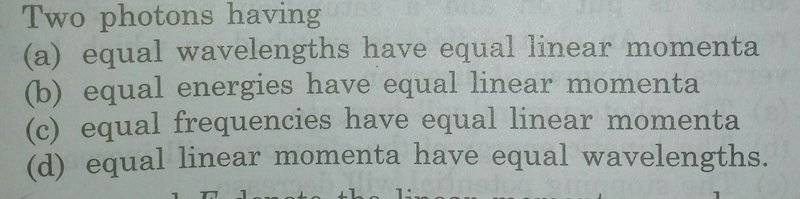# MCQ : Comparing characteristics of two photons

Jahnavi

## Homework Statement## The Attempt at a Solution

I am surely making some very silly mistake in this problem . Presuming linear momenta means magnitude of momentum , to me all four options look correct .

a) since wavelengths are same , energy is same which means momentum is same .option a) is correct .

Similarly b) , c) , d) are correct .

Or is it that the question is considering momentum as a vector (which it is ) but generally in these problems only magnitude of momentum is considered .

If this is the case , option d) is correct .

Please let me know which is correct .

#### Attachments

Homework Helper
Gold Member
2021 Award
I think the question wants you to consider momentum as a vector. Compare (a) and (d). Only one of them can be correct.

•Jahnavi
Jahnavi
I think the question wants you to consider momentum as a vector. Compare (a) and (d). Only one of them can be correct.

I think d) will be correct .

Homework Helper
Gold Member
2021 Award
I agree.

•Jahnavi
Jahnavi
In the relation E = Pc for a photon, are both the terms on RHS (momentum and speed ) treated as vectors and is it that a dot product is taken so as to get the energy term E ?

Homework Helper
Gold Member
2021 Award
In the relation E = Pc for a photon, are both the terms on RHS (momentum and speed ) treated as vectors and is it that a dot product is taken so as to get the energy term E ?
##E=pc## is a scalar equation containing scalars only. I cannot think of a situation in which the momentum and the velocity are in different directions. Can you?

Jahnavi
NoI asked because E = Pc doesn't involve vectors whereas in the OP direction of momentum vector was to be considered while relating to wavelength (energy ) .

No•